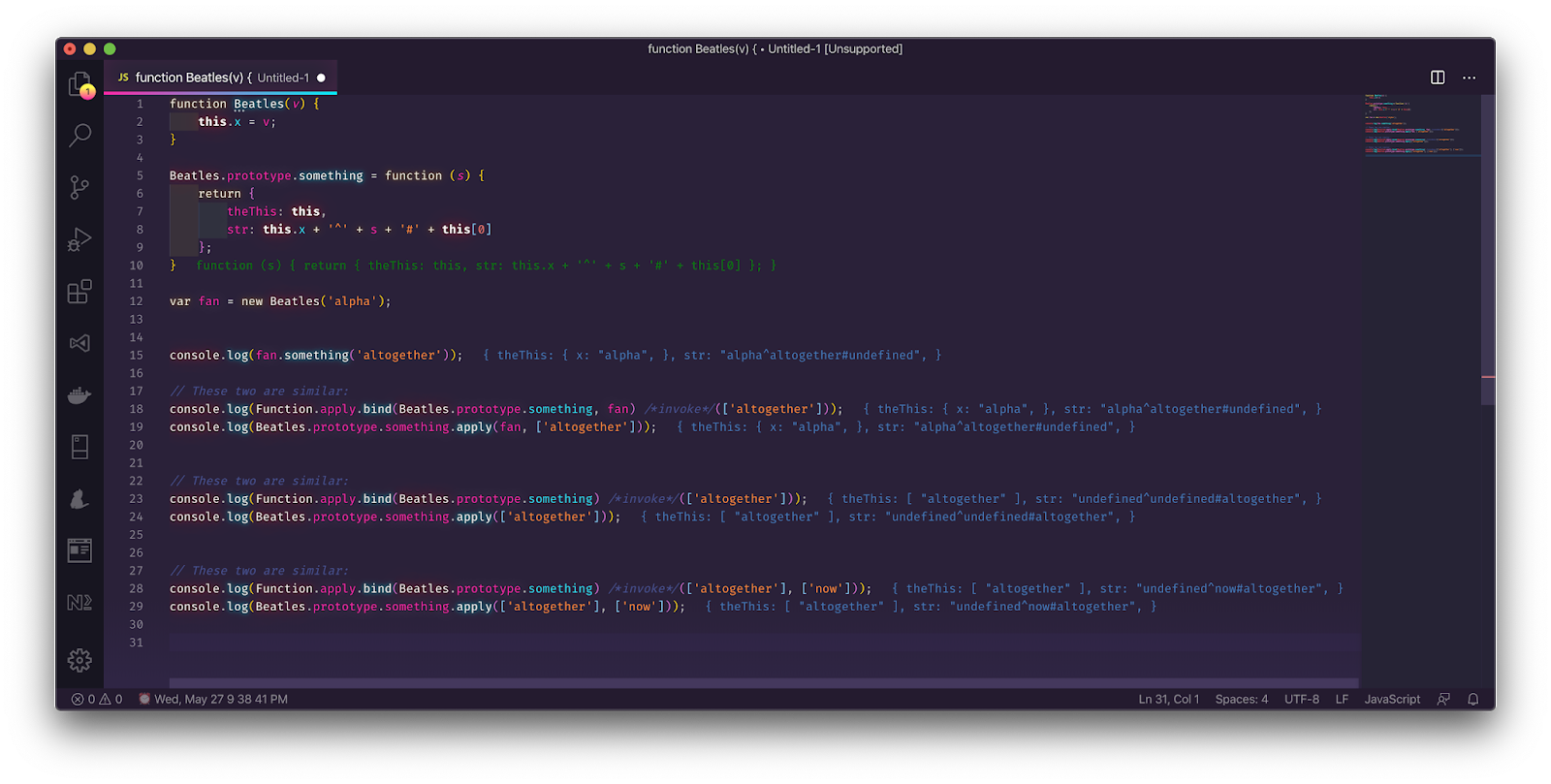## Wednesday, May 27, 2020

### .apply.bind, .call.bind

To make a shortcut for Math.max.apply(null, [5,8,6])

Use this:
```var maxArray = Function.apply.bind(Math.max, null);

var maxValue = maxArray([5,8,6]);
```

This question was asked on stackoverflow:
Why it's important to pass null for bind as argument?

Without the null on bind:
```var maxArray = Function.apply.bind(Math.max);
```

When maxArray([5,8,6]) is called, it is invoked like:

It's invoking the .apply on Math.max as:
```Math.max.apply([5,8,6]);
```

The parameter [5,8,6] is assigned to this reference.

So both maxArray([5,8,6]) and Math.max.apply([5,8,6]) are basically invoking the Math.max without any parameters, and the [5,8,6] assigned to this reference.
```Math.max();
```

We will see how .apply.bind works on our own object later and see its effect on this reference.

Both results to -Infinity:
```dev@Developers-iMac % node
Welcome to Node.js v12.14.0.
> var maxArray = Function.apply.bind(Math.max);
undefined
> maxArray([5,8,6]);
-Infinity
> Math.max.apply();
-Infinity
> Math.max();
-Infinity
>
```

We can invoke the maxArray properly like the following, but it somehow defeats the purpose of automagically passing the null on first parameter of Math.max.apply(null, [5,8,6]) expression
```dev@Developers-iMac % node
Welcome to Node.js v12.14.0.
> var maxArray = Function.apply.bind(Math.max);
undefined
> maxArray(null, [5,8,6]);
8
```

This is the result if we pass three parameters to Function.apply.bind:
```dev@Developers-iMac % node
Welcome to Node.js v12.14.0.
> var maxArray = Function.apply.bind(Math.max, null, [10, 40, 30]);
undefined
> maxArray()
40
> maxArray([50,60])
40
>
```

As for the .call, it is the same as .apply, as both them always require an object to be passed on their first parameter, which will then gets assigned to this reference, usually null is used as reference for this for utility functions. .apply is more flexible than .call, as .apply can grow its parameter, whereas .call's parameters are fixed at invocation.

Guess the output of Math.max.call(8,7,6)?

```dev@Developers-iMac % node
Welcome to Node.js v12.14.0.
> Math.max(8,7,6)
8
> Math.max.call(8,7,6)
7
> Math.max.apply(8,7,6)
Thrown:
TypeError: CreateListFromArrayLike called on non-object
> Math.max.apply([8,7,6])
-Infinity
> Math.max.apply([8,7,6],[2,4,3])
4
> Math.max.apply(null,[2,4,3])
4
> Math.max.call(null,8,7,6)
8
```

Here are other observations:Why .apply.bind requires two parameters:As we can see, if we don't pass an object reference to first parameter of .bind and we pass only one parameter, that passed parameter gets assigned to *this* reference, as seen on theThis property on line 7, likewise on this of line 8. Then the actual parameter of the method something receives nothing (undefined).